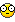## Recommended Posts

I have a script that filters out certain folders. works great.

$path = 'c:\documents and settings\'$first = FileFindFirstFile($path & '*.*')$filter = 'AdminAdministratorAllUsersdefaultuserLocalServiceNetworkService_admini$!$!svcAdmin'
$return = '' While 1$found = FileFindNextFile($first) If @error Then ExitLoop If StringInStr($filter, StringStripWS($found, 8)) Or _ Not StringInStr(FileGetAttrib($path & $found), 'D') Then ContinueLoop$return &= $found & '|' WEnd$return = StringTrimRight($return, 1) What I would like to do is to figure out a way to add or remove from the filter, and save those changes to a ini file. I'm thinking of just doing some stringinStr, but then I get lost on how to do it. What I'm doing is making a tool that: 1. looks at a list of folders and sees how old they are. 2. if the folder is a certain age, a warning message will appear. 3. You will have the option of putting that folder on a exclude list if desired so you won't see the message on account of that folder. #### Share this post ##### Link to post ##### Share on other sites I thought of a better way to do it. I will simply have a archive folder, and allow the user to move the old folders there. Keeps it simple. I'm still trying solve the issue where I get the age of each folder in the list. #### Share this post ##### Link to post ##### Share on other sites Ok, I came up with this, but it doesn't work. ???? Not sure why. It is supposed to look a group of directories, and check to see if they are a certain number of days old. If so, then I get a count of how many. Help! $TP = 0
$ini1 = "c:\" ;for testing$ini6 = 60  ;for testing
$now = _NowDate()$FileList = _FileListToArray($ini1, "*", 2) _ArrayDelete($FileList, 0)
$search_1 = _ArraySearch($FileList, "Archive", 0, 0, 0)
_ArrayDelete($FileList,$search_1)
For $q = 0 To UBound($FileList, 1)
$t = FileGetTime($FileList[$q], 1)$age = _DateDiff("D", $t,$now)
If $age >$ini6 Then $TP =$TP + 1
Next
If $TP > 0 Then MsgBox(262144 + 32 + 4, "Question", " You have " &$TP & " folders that are more than " & $ini6 & " days old." & @CRLF _ & "Would you like to move the old folders to the Archive folder?" & @CRLF _ & "" & @CRLF _ & 'Click "YES" to open the folder ' &$ini1 & ' that contains all your backups.'& @CRLF _
& 'Click "NO" to ignore this message ' & @CRLF _
& "" & @CRLF _
EndIf

##### Share on other sites

#include <Date.au3>

$path = 'c:\documents and settings\'$first = FileFindFirstFile($path & '*.*')$filter = 'AdminAdministratorAllUsersdefaultuserLocalServiceNetworkService'
$return = ''$cutoff = 180  ; cut off in days

;MsgBox(0, 'Folders found in ' & $path, _somefunc()) ; if you're interested in folders found uncomment _somefunc() Func _somefunc() While 1$found = FileFindNextFile($first) If @error Then ExitLoop If StringInStr($filter, StringStripWS($found, 8)) Or _ Not StringInStr(FileGetAttrib($path & $found), 'D') Then ContinueLoop$file_time = FileGetTime($path &$found, 1, 1)
$year = StringLeft($file_time, 4)
$month = StringMid($file_time, 5, 2)
$day = StringMid($file_time, 7, 2)
If _DateDiff('D', $year & '/' &$month & '/' & $day, @YEAR & '/' & @MON & '/' & @MDAY) >$cutoff Then _question($path &$found)
;$return &=$found & '|'  ; if you're interested in folders found uncomment
WEnd
;Return StringTrimRight($return, 1) ; if you're interested in folders found uncomment EndFunc Func _question($folder)
If MsgBox(4, 'Question', 'This following folder is old and moldy:' & @LF & $folder & @LF & 'Move it to archive folder?') = 6 Then ;DirMove($file, 'c:\path\to\your\archive\folder')  ; example of what you might want to do
MsgBox(0, '', 'Doing something with...' & @LF & $folder) EndIf EndFunc edit - One thing that is wrong with your script is: FileGetTime($FileList[$q], 1). Your$FileList array is holding folder names only. Without the paths, FileGetTime() won't work. You would need something like $t = FileGetTime($ini1 & $FileList[$q], 1).

Another problem is $now = _NowDate(),$t = FileGetTime($FileList[$q], 1) and $age = _DateDiff("D",$t, \$now). _NowDate() returns mm/dd/yyyy (unless the registry specifies something different). FileGetTime( , 1) returns YYYYMMDDHHMMSS. _DateDiff() wants "YYYY/MM/DD" for arguments.

Note how I did the date stuff in my script - you could fix your script to use it if you don't like my setup.Edited by xcal

##### Share on other sites

@xcal: This is even better than I had in mind. It is perfect! Thank you!## Create an account

Register a new account

• ### Recently Browsing   0 members

×

• Wiki

• Back

• #### Beta

• Git
• FAQ
• Our Picks
×
• Create New...